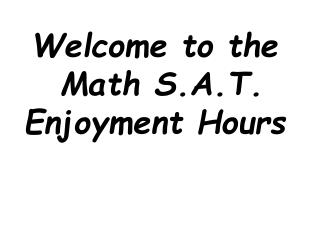# Welcome to the Math S.A.T. Enjoyment Hours - PowerPoint PPT PresentationDownload PresentationWelcome to the Math S.A.T. Enjoyment Hours

Welcome to the Math S.A.T. Enjoyment HoursDownload Presentation## Welcome to the Math S.A.T. Enjoyment Hours

- - - - - - - - - - - - - - - - - - - - - - - - - - - E N D - - - - - - - - - - - - - - - - - - - - - - - - - - -
##### Presentation Transcript

1. Welcome to the Math S.A.T. Enjoyment Hours

2. Hosted bythe B B & S Brothers Bianco, Bianco & Skeels

3. Quick Drillsky

4. #143 + 47

5. #2180 ÷ 3

6. #3145 - 96

7. #4(12)2

8. #5(2)5

9. #6(10)8

10. #7√ 169

11. #8√ (475)2

12. #9 (9)9(3)18

13. #1043 + 90 + 47

14. LET’S √ EM!

15. #143 + 47

16. #2180 ÷ 3

17. #3145 - 96

18. #4(12)2

19. #4(12)2 144

20. #5(2)5

21. #5(2)5 32

22. #6(10)8

23. #6(10)8 100,000,000

24. #7√ 169

25. #7√ 169 13

26. #8√ (475)2

27. #8√ (475)2 475

28. #9 (9)9(3)18

29. #1043 + 90 +47

30. You can have PSAT/SAT Fun everyday!Go to www.collegeboard.com

31. Strategy - ! • If the sum of 4 consecutive integers is ‘f’, then, in terms of ‘f’, what is the least of these integers? • A) f/4 B) (f - 2)/4 C) (f - 3)/4 D) (f - 4)/4 E) (f - 6)/4

32. Strategy - Substitute! • If the sum of 4 consecutive integers is ‘f’, then, in terms of ‘f’, what is the least of these integers? • A) f/4 B) (f - 2)/4 C) (f - 3)/4 D) (f - 4)/4 E) (f - 6)/4

33. Strategy - sdrawkcaB kroW • Work backwards!!!! Fill in the answer choices for complex algebra problems. • Example: If (a/2)3 = a2, a≠0, then a = • A) 2 B) 4 C) 6 D) 8 E) 10 *From last lesson - ran out of time!

34. Helpful Hint: • Remember the answer choices are arranged from least to greatest so it may help start in the middle and proceed in the right direction.

35. Objectives: • To review Geometry concepts on SAT. • To introduce Student Produced Response problems.(SPR) • To introduce 1 more strategy.

36. GEOMETRY & MATH • WE ALL KNOW FIGURES INVOLVED IN GEOMETRY

37. GEOMETRY & MATH • WE ALL KNOW FIGURES INVOLVED IN GEOMETRY

38. GEOMETRY & MATH • WE ALL KNOW FIGURES INVOLVED IN GEOMETRY

39. GEOMETRY & MATH • BUT WITH A FEW DEFINITIONS WE CAN TACKLE MANY PROBLEMS WHICH OTHERWISE WOULD BE IMPOSSIBLE

40. ESSENTIALS OF GEOMETRY • A RIGHT ANGLE:

41. ESSENTIALS OF GEOMETRY • A RIGHT ANGLE: An angles with a measure of 90°

42. ESSENTIALS OF GEOMETRY • AN ACUTE ANGLE:

43. ESSENTIALS OF GEOMETRY • AN ACUTE ANGLE: An angle which measurement is less than 90°

44. ESSENTIALS OF GEOMETRY • AN OBTUSE ANGLE:

45. ESSENTIALS OF GEOMETRY • AN OBTUSE ANGLE: An angle which measurement is more than 90°